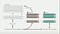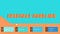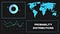Artificial Intelligence and Data Science Enthusiast. Updating Neural Network parameters since 2002.

# What is Classification Problem ?

In general , Supervised Learning consists of 2 types of problem setting.

• Regression : It is the type of problem where the data scientist models the relationship between the independent variables and the continuous dependent variable using a suitable model and used that to give accurate predictions for future input data.
For example,
Predicting the sales of Ice cream on a given day, given the temperature.
Here, Sales of Ice Cream is a continuous variable which means it can take any value.
eg: 500, 100, 10,000, 52,123, 931…etc.
• Classification: It is a type of problem where the data scientist has…

# Cross Validation - What, Why and How | Machine Learning

## What is Cross Validation , Why it is used and What are the different types ?Cross Validation Cycle

# What is Cross Validation and Why do we Need it ?

In a Supervised Machine Learning problem , we usually train the model on the dataset and use the trained model to predict the target, given new predictor values.
But, How do we know if the model we have trained on the dataset will producing effective and accurate results on the new input data.
We cannot conclude that the model has performed well based on the error rates or certain statistic measures (such as R square statistic) that we get from the dataset on which the model is trained.
The main problem is that there is no way of knowing if the model…

# What is Synergy Effect in Linear Regression | Machine Learning

Synergy Effect or Interaction Effect is a phenomenon that arises in the multiple linear regression setting in machine learning, when increase in the value of one Independent variable increases the impact of another Independent variable on the dependent Variable.
It’s okay if this above statement is not easily understandable. Let’s look at an example
Note: You should be familiar with multiple linear regression to understand this

# Advertisement Sales Data-set

Let’s consider a data-set with advertising budget of a company for different categories and the sales of the company. So, the 3 columns of the data-set are Radio Advertising, Newspaper Advertising and Sales.
Let’s…

# Introduction To Ensemble Learning | Optimal Machine LearningCreated by ashwinhprasad with canva

When it comes to predictive modeling , A single algorithmic model might be not be enough to make the most optimal predictions.
One of the most effective methodologies in machine learning is Ensemble Modeling or Ensembles.

Ensemble Modeling is the combination of multiple machine learning models that follow the same or different algorithm to make better overall predictions.
It is usually these types of models that win the machine learning competitions conducted by netflix or kaggle.

# Categories

Ensemble modeling methods can be split into various categories.
they are:

1. Sequential

# A Quick Look Into Probability DistributionsProbability Distribution Thumbnail

# What is a Probability Distribution ?

Probability Distribution : A probability Distribution shows the list of probabilities associated with each value or a range of values for a discrete or a continuous random variable.
Based on the Type of Random Variable, Probability Distributions can be split into Continuous Probability Distributions and Discrete Probability Distributions

# What are Convolutional Neural Networks ?

Convolutional Neural Networks is a type of neural network that is mostly used for Image Datasets and The shared parameters feature of convolutional network reduces the number of parameters for the model and this also makes it efficient in pattern Edge-Detection. This blog post contains the implementation of Convolutional Neural Networks with tensorflow 2.0 on the Cats vs Dogs dataset

# About the Dataset

Cats vs Dogs dataset is one of the most famous dataset used in the field of machine learning, which was developed by the partnership between pathfinder.com and Microsoft.

The Dataset is available at : https://www.microsoft.com/en-us/download/details.aspx?id=54765

Before we go into creating…

# Tensorflow 2 for DeepLearning - Artificial Neural Networks

Note: The code files will be available at : https://github.com/ashwinhprasad/Tensorflow-2.0/blob/master/TF2-(4)-ANN/TF2-ANNs.ipynb

# Introduction

Artificial Neural Networks are the traditional neural networks which means that there are more than or equal to one layer between the input and the output layer. This allows for the model to adapt for the non linearity and form complex functions which makes it useful in real life.

This blog post does not covers only the implementation of feed forward or artificial neural network with tensorflow 2 and not the theory part of the artificial neural network.

# Tensorflow 2 for Deep Learning — Logistic Regression ( Softmax )

Note : The Program files for tensorflow 2 can be found on - https://github.com/ashwinhprasad/Tensorflow-2.0

Logistic Regression is used for Classification tasks and This Blog will take you through the implementation of logistic regression using Tensorflow 2. This blog post won’t be covering about the theories regarding logistic regression and theory is a pre-requisite.

# Let’s jump to the code part :

## 1. Importing The Dataset

The Dataset that is used in this example is iris dataset from the sklearn library.
we are importing the dataset and storing it in the form of a pandas dataframe

`#importing the librariesimport numpy as npimport tensorflow as tfimport pandas as pdimport matplotlib.pyplot as pltimport seaborn as…`

# Tensorflow 2 for Deep Learning -Linear Regression

Code files will be available at : https://github.com/ashwinhprasad/Tensorflow-2.0

# What is Linear Regression ?

Linear regression is basically using a equation of a line to find out the linear relationship between 2 variables. By finding the linear relationship, I mean finding the parameters ( Slope and Intercept).

y = m*x + c
y : dependent variable
x : independent variable
m : slope
c : intercept

# TensorFlow 2 — Common Functions

Note : All code files will be available at https://github.com/ashwinhprasad/Tensorflow-2.0
This blog post will cover some basic functions that will be repeatedly used a lot in tensorflow 2.

# Random

random.normal generates random values of the given shape, which follow normal distribution
and random.uniform generates random values in such a way that probability of choosing any number from the random bunch is almost uniform

`#normal distributionx1 = tf.random.normal(shape=(5,5),mean=0,stddev=1) #normal distributionprint(x1)output: tf.Tensor([[-1.1473149e+00  5.1616412e-01 -2.8656033e-01 -1.4161720e-03  -6.7782238e-02] [ 1.5549400e-01 -1.8609362e+00  7.8299832e-01 -7.3712116e-01  -3.0330741e-01] [ 5.6524660e-02  1.0138390e-01  1.2218195e+00  1.2505690e+00   3.0457941e-01] [ 3.6436683e-01 -8.6699528e-01…`